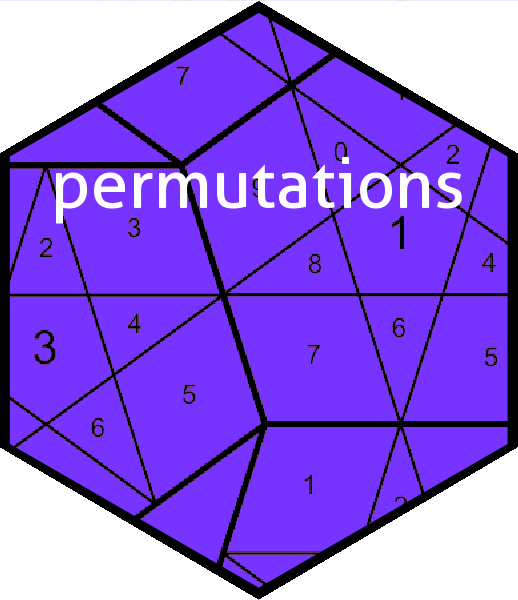# Introduction

We will consider $$S_9$$, the symmetric group on 9 elements, and representations of its elements. First we will load the package and change the default print method:

library("permutations")
options("print_word_as_cycle" = FALSE)

Now we will generate a couple of permutations, a and b:

a <- as.word(char2cycle("(175296)(348)"))
b <- as.word(char2cycle("(27)(45)(89)"))
a
#>     {1} {2} {3} {4} {5} {6} {7} {8} {9}
#>  7   9   4   8   2   1   5   3   6
b
#>     {1} {2} {3} {4} {5} {6} {7} {8} {9}
#>  .   7   .   5   4   .   2   9   8

Now we will show a representation of $$S_9$$, using permutation matrices:

M <- diag(9)
rownames(M) <- 1:9
colnames(M) <- 1:9
M
#>   1 2 3 4 5 6 7 8 9
#> 1 1 0 0 0 0 0 0 0 0
#> 2 0 1 0 0 0 0 0 0 0
#> 3 0 0 1 0 0 0 0 0 0
#> 4 0 0 0 1 0 0 0 0 0
#> 5 0 0 0 0 1 0 0 0 0
#> 6 0 0 0 0 0 1 0 0 0
#> 7 0 0 0 0 0 0 1 0 0
#> 8 0 0 0 0 0 0 0 1 0
#> 9 0 0 0 0 0 0 0 0 1

We will use the map $$\phi\colon S_9\longrightarrow\operatorname{Aut}\left(\mathbb{R}^9\right)$$ given by $$\phi(a)=$$ M[a,]. Then

M[a,]
#>   1 2 3 4 5 6 7 8 9
#> 7 0 0 0 0 0 0 1 0 0
#> 9 0 0 0 0 0 0 0 0 1
#> 4 0 0 0 1 0 0 0 0 0
#> 8 0 0 0 0 0 0 0 1 0
#> 2 0 1 0 0 0 0 0 0 0
#> 1 1 0 0 0 0 0 0 0 0
#> 5 0 0 0 0 1 0 0 0 0
#> 3 0 0 1 0 0 0 0 0 0
#> 6 0 0 0 0 0 1 0 0 0

permutes the rows of $$M$$ with permutation a. Note how the row names are permuted as well as the elements of $$M$$. Verifying that $$\phi$$ is indeed a homomorphism—that is, $$\phi(\iota)=I_9$$ and $$\phi(a)\phi(b)=\phi(a\circ b)$$—is straightforward:

all(M[as.word(id,9),] == diag(9))
#>  TRUE
all(M[a*b,] == M[a,] %*% M[b,])
#>  TRUE

In the second line above, note that the left hand side of the equality is group composition, while the right hand side is matrix multiplication. We can further verify that $$\phi\left(a^{-1}\right)=\phi(a)^{-1}$$:

all(M[inverse(a),] == solve(M[a,]))
#>  TRUE

again with group inversion on the left and matrix inversion on the right.

The map $$\psi(a)=$$ M[,a] is not a homomorphism:

all(M[,a*b] == M[,a] %*% M[,b])
#>  FALSE

but we can “rescue” it by considering a group operation $$\star$$ defined by $$a\star b=b\circ a$$:

all(M[,b*a] == M[,a] %*% M[,b])
#>  TRUE